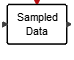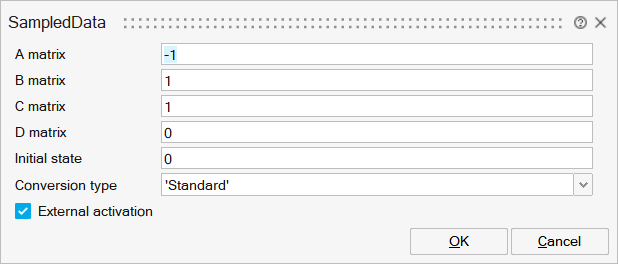# SampledData

This block implements a sampled data system. The system is defined by the block parameters, which are the A,B,C,D matrices of the underlying continuous-time linear system and the associated initial state vector. The resulting discrete-time linear system is computed using the values of the parameters and the activation period of the block. The block must be activated by a periodic activation signal.Dynamical

## Description

This block implements a sampled data system. The system is defined by the block parameters, which are the A,B,C,D matrices of the underlying continuous-time linear system and the associated initial state vector. The resulting discrete-time linear system is computed using the values of the parameters and the activation period of the block. The block must be activated by a periodic activation signal.

## ParametersNameLabelDescriptionData TypeValid Values

A

A matrix

real nxn matrix where n is the number of states, default value: 1.

Matrix

B

B matrix

real nxm matrix where n is the number of states and m is the number of inputs, default value: 1. B=[ ] if block has no input.

Matrix

C

C matrix

real rxn matrix, default value: 1. r is the number of outputs and n is the number of states.

Matrix

D

D matrix

real rxm matrix where r is the number of outputs and m is the number of inputs, default value: 1.

Matrix

x0

Initial state

vector of size n or scalar (expanded to a vector of size n).

Vector

discmethod

Conversion type

Discretization method.

String

'Standard'
'Bilinear'

externalActivation

External activation

This parameter defines if the block receives an external activation or inherits its activation through its regular input ports. When External Activation is used, an activation port is added to the block. The block should be activated by a periodic activation signal.

Number

0
1

## Ports

NameTypeDescriptionIO TypeNumber

Port 1

explicit

output

1

Port 2

explicit

input

1

Port 3

activation

input

externalActivation

NameValueDescription

always active

no

direct-feedthrough

no, except when D is not zero

zero-crossing

no

mode

no

continuous-time state

no

discrete-time state

yes